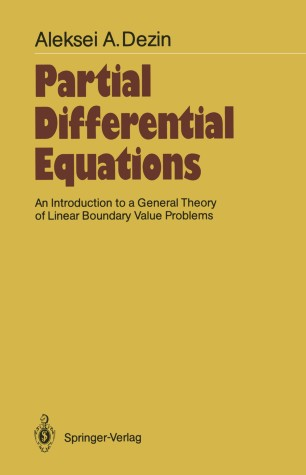# Partial Differential Equations

## An Introduction to a General Theory of Linear Boundary Value Problems

• Aleksei A. DezinBook

Part of the Springer Series in Soviet Mathematics book series (SSSOV)

1. Front Matter
Pages I-XII
2. Aleksei A. Dezin
Pages 1-23
3. Aleksei A. Dezin
Pages 24-54
4. Aleksei A. Dezin
Pages 55-68
5. Aleksei A. Dezin
Pages 69-80
6. Aleksei A. Dezin
Pages 81-104
7. Aleksei A. Dezin
Pages 105-118
8. Aleksei A. Dezin
Pages 119-130
9. Aleksei A. Dezin
Pages 131-143
10. Aleksei A. Dezin
Pages 144-145
11. Back Matter
Pages 146-165

### Introduction

Let me begin by explaining the meaning of the title of this book. In essence, the book studies boundary value problems for linear partial differ­ ential equations in a finite domain in n-dimensional Euclidean space. The problem that is investigated is the question of the dependence of the nature of the solvability of a given equation on the way in which the boundary conditions are chosen, i.e. on the supplementary requirements which the solution is to satisfy on specified parts of the boundary. The branch of mathematical analysis dealing with the study of boundary value problems for partial differential equations is often called mathematical physics. Classical courses in this subject usually consider quite restricted classes of equations, for which the problems have an immediate physical context, or generalizations of such problems. With the expanding domain of application of mathematical methods at the present time, there often arise problems connected with the study of partial differential equations that do not belong to any of the classical types. The elucidation of the correct formulation of these problems and the study of the specific properties of the solutions of similar equations are closely related to the study of questions of a general nature.

### Keywords

Boundary value problem differential equation mathematical analysis partial differential equation

#### Authors and affiliations

• Aleksei A. Dezin
• 1
1. 1.Steklov Mathematical InstituteMoscowUSSR

### Bibliographic information

• DOI https://doi.org/10.1007/978-3-642-71334-7
• Copyright Information Springer-Verlag Berlin Heidelberg 1987
• Publisher Name Springer, Berlin, Heidelberg
• eBook Packages
• Print ISBN 978-3-642-71336-1
• Online ISBN 978-3-642-71334-7
• Series Print ISSN 0939-1169
• Buy this book on publisher's site